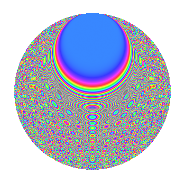# Properties

 Label 672.2.cfLevel 672 Weight 2 Character orbit cf Rep. character $$\chi_{672}(19,\cdot)$$ Character field $$\Q(\zeta_{24})$$ Dimension 512 Newform subspaces 1 Sturm bound 256 Trace bound 0

# Related objects

## Defining parameters

 Level: $$N$$ $$=$$ $$672 = 2^{5} \cdot 3 \cdot 7$$ Weight: $$k$$ $$=$$ $$2$$ Character orbit: $$[\chi]$$ $$=$$ 672.cf (of order $$24$$ and degree $$8$$) Character conductor: $$\operatorname{cond}(\chi)$$ $$=$$ $$224$$ Character field: $$\Q(\zeta_{24})$$ Newform subspaces: $$1$$ Sturm bound: $$256$$ Trace bound: $$0$$

## Dimensions

The following table gives the dimensions of various subspaces of $$M_{2}(672, [\chi])$$.

Total New Old
Modular forms 1056 512 544
Cusp forms 992 512 480
Eisenstein series 64 0 64

## Trace form

 $$512q + O(q^{10})$$ $$512q + 64q^{14} + 8q^{16} - 8q^{18} - 16q^{22} - 16q^{23} - 40q^{28} + 48q^{35} + 32q^{43} + 8q^{44} + 48q^{50} - 72q^{52} + 32q^{53} + 32q^{58} - 192q^{59} - 48q^{60} + 144q^{64} - 144q^{66} - 16q^{67} - 72q^{70} + 128q^{71} + 32q^{74} + 48q^{78} - 72q^{80} - 120q^{82} + 88q^{88} - 48q^{91} + 128q^{92} - 72q^{94} - 120q^{96} - 136q^{98} + O(q^{100})$$

## Decomposition of $$S_{2}^{\mathrm{new}}(672, [\chi])$$ into newform subspaces

Label Dim. $$A$$ Field CM Traces $q$-expansion
$$a_2$$ $$a_3$$ $$a_5$$ $$a_7$$
672.2.cf.a $$512$$ $$5.366$$ None $$0$$ $$0$$ $$0$$ $$0$$

## Decomposition of $$S_{2}^{\mathrm{old}}(672, [\chi])$$ into lower level spaces

$$S_{2}^{\mathrm{old}}(672, [\chi]) \cong$$ $$S_{2}^{\mathrm{new}}(224, [\chi])$$$$^{\oplus 2}$$

## Hecke characteristic polynomials

There are no characteristic polynomials of Hecke operators in the database